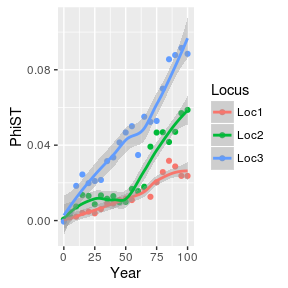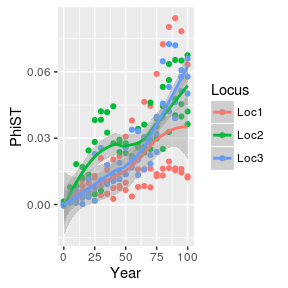# More on simulating landscapes

The philosophy of rmetasim is to provide flexible and powerful ways to simulate realistic demography underlying population genetics. Usually when a software author uses the words flexible'' andpowerful’‘, it usually means hard to use’’. rmetasim has proven to be a bit impenetrable to users starting off so, empirically, this software fits into that category. This vignette is an attempt to explain some of the approaches to simulation that rmetasim can help implement, and lower the activation energy required for its use.

## Alternatives to landscape.simulate()

The main simulation function, is the typical approach to simulations in rmetasim. Each simulation step (year) is composed of several substeps:

1. choose populations for extirpation and if any, kill them off
2. reproduce
3. impose survival and growth to new stages
4. reduce populations to the carrying capacity

These steps are implemented n C++ for speed. It is possible, however, to run each of these steps separately. This can help in debugging a landscape or implementing a simulation where survival precedes reproduction, or changing the time in which extinction occurs or just for fun.

Here is a simulation using landscape.simulate()

library(rmetasim)
rland <- landscape.new.example()
rland <- landscape.simulate(rland,10)
landscape.amova(rland)
##        Phi        Phi        Phi
## 0.06613830 0.12350636 0.02631133

Here is the same simulation with the individual steps implemented in R functions (which call corresponding C++ functions)

rland <- landscape.new.example()
for (gen in 1:10)
{
rland <- landscape.extinct(rland)
rland <- landscape.reproduce(rland)
rland <- landscape.survive(rland)
rland <- landscape.carry(rland)
}
landscape.amova(rland)
##        Phi        Phi        Phi
## 0.01081319 0.16664878 0.03271328

### or with magrittr

library(magrittr)
rland <- landscape.new.example()
for (gen in 1:10)
{
rland <- rland %>% landscape.extinct() %>%  landscape.reproduce() %>%
}
landscape.amova(rland)
##         Phi         Phi         Phi
## 0.009046521 0.064582402 0.032305609

These approaches are a little slower because there is conversion between the R version of a landscape (a list) and the internal C++ backend representation more often.

## Examining temporal dynamics

You can see in the previous section how the same landscape can be the input and output of a simulation. You can use this with loops to examine the temporal dynamics of a simulation through time.

The following runs a simulation for 100 years and checks the among-population differentiation every 5 years. Finally it plots the results. This landscape has no migration among the two populations, so the among-pop differentiation should be an increasing function, with some potentially interesting patterns.

In general it is advisable to make the rmetasim sessions less script-like and more a set of functions. The following is a new function to create landscapes.

create.land <- function()
{
### CREATE A LANDSCAPE
###first set up the matrices for local demographies
S <- matrix(c(0, 0, 1, 0), byrow = TRUE, nrow = 2)
R <- matrix(c(0, 1.1, 0, 0), byrow = TRUE, nrow = 2)
M <- matrix(c(0, 0, 0, 1), byrow = TRUE, nrow = 2)

#and epochs
S.epoch <- matrix(rep(0, 36), nrow = 6)
R.epoch <- matrix(rep(0, 36), nrow = 6)
M.epoch <- matrix(rep(0, 36), nrow = 6)

##now create the landscape
landscape.new.empty() %>%
landscape.new.intparam(h=3,s=2) %>%
landscape.new.switchparam(mp=0) %>%
landscape.new.floatparam() %>%
landscape.new.local.demo( S, R, M) %>%
landscape.new.epoch(S = S.epoch, R = R.epoch, M = M.epoch,
carry = c(1000, 1000, 1000)) %>%
landscape.new.locus(type = 1, ploidy = 2,
mutationrate = 0.001, transmission = 0, numalleles = 5) %>%
landscape.new.locus(type = 0, ploidy = 1,
mutationrate = 0.005, numalleles = 3, frequencies = c(0.2, 0.2, 0.6)) %>%
landscape.new.locus(type = 2, ploidy = 1,
mutationrate = 0.007, transmission = 1, numalleles = 3,
allelesize = 75) %>%
landscape.new.individuals(rep(c(500,500),3))
}

Now this function is used in the experiment described above

rland <- create.land()
retval <- matrix(0,ncol=4,nrow=21) #store the results col1 = gen, cols2-3 PhiST
retval[1,] <- c(0,landscape.amova(rland)) #before any evolution occurs. Both populations from same source
for (i in 2:21)
{
rland <- landscape.simulate(rland,5)
retval[i,] <- c(rland$intparam$currentgen,landscape.amova(rland))
}
###the rest is just plotting the matrix of PhiSTs
###you can use any kind of graphics, of course.  using ggplot2 here.
colnames(retval) <- c("gen","Loc1","Loc2","Loc3")
library(ggplot2)
library(reshape2)
pl <- ggplot(melt(as.data.frame(retval),measure.vars=c("Loc1","Loc2","Loc3")),
aes(x=gen,y=value,group=variable,color=variable))
pl <- pl + labs(y="PhiST",x="Year",color="Locus")
pl <- pl + geom_point() + geom_smooth()
pl### Replicating this “experiment”

The graph in the previous section may be a little bit interesting. Does it hold up over multiple replicates? The following code replicates the previous experiment 3 times, a more useful number of replicates for understanding these time-series would be much higher (30? 100?). The data are then plotted like before.

The starting point is the same landscape creation function in the previous section.

number.reps=3
retlst <- lapply(1:number.reps,function(x)
{
rland <- create.land()
retval <- matrix(0,ncol=5,nrow=21)
retval[1,] <- c(0,landscape.amova(rland),x)
for (i in 2:21)
{
rland <- landscape.simulate(rland,5)
retval[i,] <- c(rland$intparam$currentgen,landscape.amova(rland),x)
}
colnames(retval) <- c("gen","Loc1","Loc2","Loc3","replicate")
retval
})
retdf <- as.data.frame(do.call(rbind,retlst))

pl <- ggplot(melt(retdf,measure.vars=c("Loc1","Loc2","Loc3")),
aes(x=gen,y=value,group=variable,color=variable))
pl <- pl + labs(y="PhiST",x="Year",color="Locus")
pl <- pl + geom_point() + geom_smooth()
plUsing lapply here is really useful because it parallelizes well using routines from the parallel’’ package.